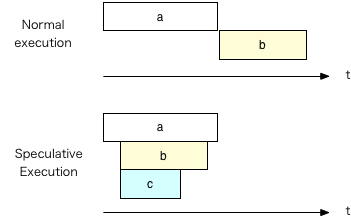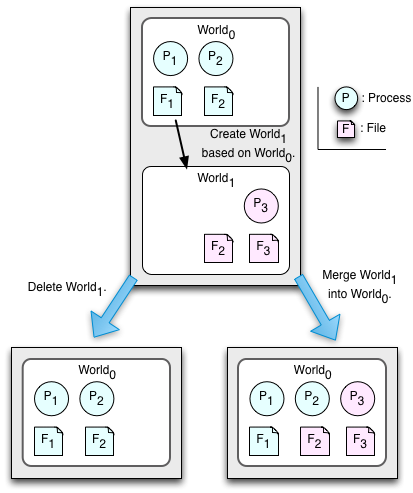# \$B%H%i%s%6%/%7%g%s!"(BArgus\$B!"Ej5!E*!&3Z4QE*

```\$BJB9T%7%9%F%`(B

\$B%7%9%F%`>pJs7O>pJs9)3X0h(B,
\$B%7%9%F%`>pJs9)3X8&5f2J%3%s%T%e!<%?%5%\$%(%s%9@l96(B
\$B?7>k(B \$BLw(B
<yas@cs.tsukuba.ac.jp>
```

\$B\$3\$N%Z!<%8\$O!" ``` http://www.cs.tsukuba.ac.jp/~yas/cs/csys-2013/2013-12-10 ```
\$B\$"\$k\$\$\$O!" ` http://www.cs.tsukuba.ac.jp/~yas/cs/`
` http://www.cs.tsukuba.ac.jp/~yas/`

## \$B"#O"Mm;v9`(B

• \$B4|Kv;n83\$O9T\$\$\$^\$;\$s!#4|Kv%l%]!<%H\$b\$"\$j\$^\$;\$s!#Kh2s\$N%l%]!<%H\$G@.(B \$B@S\$rIU\$1\$^\$9!#(B There is no final exam and no final report. Grade are determined by individual class reports.
• \$B%l%]!<%H=P\$7\$F\$\$\$J\$\$?M\$O(B Web \$B%Z!<%8\$+\$i=P\$7\$F\$/\$@\$5\$\$!#(B You must submit reports via the Web page.
• \$B%l%]!<%H\$O!"(B12\$B7n(B15\$BF|(B \$B\$^\$G\$KA4ItDs=P\$7\$F\$/\$@\$5\$\$!#(B You must submit all the reports by December 15.
• \$B\$\$\$/\$D\$+\$N%l%]!<%H\$O:FDs=P\$NI,MW\$,\$"\$k\$+\$b\$7\$l\$^\$;\$s!#(B You may have some reports to post again.

## \$B"#:#F|\$N=EMW\$JOC(B

\$B#2\$D\$NAj(B
\$BBh#1Aj(B
\$BA4%W%m%;%9\$r(B Commit \$B\$b(B Abort \$B\$b\$G\$-\$k>uBV\$K\$9\$k!#(B
1. \$B%3!<%G%#%M!<%?\$O!"%m%0\$K!V=`Hw!W\$H=q\$/!#(B
2. \$B%3!<%G%#%M!<%?\$O!"%5%V%*!<%G%#%M!<%H\$K!V=`Hw!W%a%C%;!<%8\$rAw\$k!#(B
3. \$B%5%V%*!<%G%#%M!<%H\$O!"%m%0\$K!V=`Hw40N;!W\$H=q\$/!#(B
4. \$B%5%V%*!<%G%#%M!<%H\$O!"%3!<%G%#%M!<%?\$K!V=`Hw40N;!W%a%C%;!<%8\$rAw\$k!#(B
5. \$B%3!<%G%#%M!<%?\$O!"A4\$F\$N%5%V%*!<%G%#%M!<%H\$+\$i\$N1~Ez\$rBT\$D!#(B
\$BBh#2Aj(B
\$B
• \$B%3!<%G%#%M!<%?\$O!"%m%0\$K!V%3%_%C%H!W\$H=q\$/!#(B
• \$B%3!<%G%#%M!<%?\$O!"%5%V%*!<%G%#%M!<%H\$K!V%3%_%C%H!W%a%C%;!<%8\$rAw\$k!#(B
• \$B%5%V%*!<%G%#%M!<%H\$O!"%m%0\$K!V%3%_%C%H40N;!W\$H=q\$/!#(B
• \$B%5%V%*!<%G%#%M!<%H\$O!"%3!<%G%#%M!<%?\$K!V%3%_%C%H40N;!W%a%C%;!<%8\$rAw\$k!#(B
• \$B%3!<%G%#%M!<%?\$O!"A4\$F\$N%5%V%*!<%G%#%M!<%H\$+\$i\$N1~Ez\$rBT\$D!#(B
• \$B%/%i%C%7%e\$7\$?;~\$K\$O!"%m%0\$+\$i2sI|\$5\$;\$k!#(B

## \$B"#(BArgus

\$B%H%i%s%6%/%7%g%s5!G=(B\$B\$rFbIt\$K4^\$`8@8l!#(B \$BH/2;\$O!"1Q8lFI\$_\$G%"!<%,%9!#%.%j%7%c?@OCFI\$_\$G\$O!"%"%k%4%9!#(B

Barbara Liskov: "Distributed programming in Argus", Communications of the ACM, Vol.31, No.3, pp.300-312, 1988. `http://dl.acm.org/citation.cfm?id=42399`

\$BJ8K!\$O!"(BCLU \$B8@8l\$K;w\$F\$\$\$k!#(B

\$B;29M(B:

### \$B"!%,!<%G%#%"%s(B(guardian)

\$BJB9T\$KF0:n\$9\$k%*%V%8%'%/%H(B(\$B8=:_\$NMQ8l\$G\$O%9%l%C%I(B)\$B\$r
• \$B%O%s%I%i(B(handler)\$B\$H8F\$P\$l\$k(B \$BB>\$N%,!<%G%#%"%s\$+\$i8F\$V\$3\$H\$,\$G\$-\$k \$B=i4|2=MQ\$K%/%j%(!<%?(B(creator)\$B\$H8F\$P\$l\$k%(%s%H%j\$r;}\$D!#(B

### \$B"!0BDj\$JJQ?t(B(Stable variable)

\$B%,!<%G%#%"%s\$O!"#2
\$B0BDj(B(stable)
\$B%/%i%C%7%e!J:F5/F0!K\$7\$F\$b!"@8\$-;D\$k!#(B
\$B4xH/(B(volatile)
\$B%/%i%C%7%e!J:F5/F0!K\$9\$k\$H!"CM\$,<:\$o\$l\$k!#(B

### \$B"!%"%/%7%g%s(B(action)

\$B0BDj\$JJQ?t\$KBP\$9\$k%H%i%s%6%/%7%g%s\$ruBV\$+\$i!"uBV\$XA+0\\$5\$;\$k!#(B( \$BESCf\$G%/%i%C%7%e\$7\$?\$i!"85\$KLa\$9!#(B)

\$B%H%C%W%"%/%7%g%s(B
\$B%H%C%W%l%Y%k\$N%H%i%s%6%/%7%g%s\$r \$B%5%V%"%/%7%g%s(B
\$B%M%9%H\$7\$?%H%i%s%6%/%7%g%s\$r \$BB>\$N%,!<%G%#%"%s\$N%O%s%I%i\$N8F=P\$7(B(\$B1s3V\$B"!(BArgus\$B\$K\$*\$1\$k%H%i%s%6%/%7%g%s\$N
• \$B#2Aj%3%_%C%H(B
• \$B=q9~\$_%m%C%/\$G!":n6HMQ\$N%3%T!<\$,:n\$i\$l\$k!#(B

### \$B"!%,!<%G%#%"%s\$N@k8@(B/Declaration of guardians

1. \$BL>A0(B Name
2. \$B@8@.%k!<%A%s!#0z?t\$N \$B0BDj\$JJQ?t!#(BStable variables
3. \$B4xH/E*\$JJQ?t!#(BVolatile variables
4. \$B%O%s%I%i \$B%P%C%/%0%i%s%I!&%k!<%A%s!#%,!<%G%#%"%s<+?H\$N%W%m%0%i%`!#(B Backgroud routines.
5. \$BI|5luBV\$KLa\$9!#(B Recovery procedures.
\$B0BDj\$JJQ?t\$K\$D\$\$\$F\$O!"%7%9%F%`\$,<+F0E*\$K@09g@-\$,uBV\$KI|5l\$9\$k!#(B

### \$B"!6d9T\$N;YE9(B/A bank branch in Argus

```   1: branch = guardian is create handles total, open, close, deposit, withdraw
2:   % type definitions
3:   htable = atomic_array[bucket]
4:   bucket = atomic_array[pair]
5:   pair = atomic_record[num: account_number, acct: acct_info]
6:   acct_info = atomic_record[bal: int]
7:   account_number = atomic_record[code: string, num: int]
8:   intcell = atomic_record[val: int]
9:
10:   stable ht: htable    % the table of accounts
11:   stable code: string  % the code for the branch
12:   stable seed: intcell % the seed for generating new account numbers
13:
14:   create = creator (c: string, size: int) returns (branch)
15:     code := c
16:     seed.val := 0
17:     ht := htable\$new()
18:     for i: int in int\$from_to(1, size) do
20:     end
21:     return (self)
22:   end create
23:
24:   total = handler () returns (int)
25:     sum: int := 0
26:     for b: bucket in htable\$elements(ht) do
27:       for p: pair in bucket\$elements(b) do
28:         sum := sum + p.acct.bal
29:       end
30:     end
31:     return (sum)
32:   end total
33:
34:   open = handler () returns (account_number)
35:     intcell\$write_lock(seed) % get a write lock on the seed
36:     a: account_number := account_number\${code: code, num: seed.val}
37:     seed.val := seed.val + 1
38:     bucket\$addh(ht[hash(a.num)], pair\${num: a, acct: acct_info\${bal: 0}})
39:     return (a)
40:   end open
41:
42:   close = handler (a: account_number) signals (no_such_acct, positive_balance)
43:     b: bucket := ht[hash(a.num)]
44:     for i: int in bucket\$indexes(b) do
45:       if b[i].num != a then continue end
46:       if b[i].acct.bal > 0 then signal positive-balance end
47:       b[i] := bucket\$top(b) % store topmost element in place of closed account
48:       bucket\$remh(b) % discard topmost element
49:       return
50:     end
51:     signal no_such_acct
52:   end close
53:
54:   lookup = proc (a: account_number) returns (acct_info) signals (no_such__acct)
55:     for p: pair in bucket\$elements(ht[hash(a.num)]) do
56:       if p.num = a then return (p.acct) end
57:     end
58:     signal no_such_acct
59:     end lookup
60:
61:   deposit = handler (a: account_number, amt: int) signals (no_such_acct,
62:                                                                 negativ_amount)
63:     if amt < 0 then signal negative_amount end
64:     ainfo: acct_info := lookup(a) resignal no_such_acct
65:     ainfo.bal := ainfo.bal + amt
66:   end deposit
67:
68:   withdraw = handler (a: account_number, amt: int) signals (no_such_acct,
69:                                          negative_amount, insufficient_funds)
70:     if amt < 0 then signal negative amount end
71:     ainfo: acct_info := lookup(a) resignal no_such_acct
72:     if ainfo.bal < amt then signal insufficient_funds end
73:     ainfo.bal := ainfo.bal - amt
74:   end withdraw
75: end branch
```
• \$B%,!<%G%#%"%s\$rDj5A\$7!"(Bbranch \$B\$H\$\$\$&JQ?t\$K%P%\$%s%I(B
• \$B%/%j%(!<%?\$O!"(Bcreate()
• \$B%O%s%I%i\$O!"(Btotal(), open(), close(), deposit(), withdraw()
• \$B7?Dj5A!#(Brecord \$B\$O!"9=B\$BN\$NDj5A!#(B
• atomic \$B\$J\$N\$G!"%7%9%F%`\$K\$h\$j%H%i%s%6%/%7%g%s\$NBP>]\$H\$J\$k!#(B
• \$BJQ?tDj5A!#(Bstable \$B\$J\$N\$G!"A4It0BDj!#(B \$B4xH/E*\$JJQ?t\$,\$J\$\$\$N\$G!"%/%i%C%7%e\$+\$i\$N2sI|%3!<%I\$O\$J\$\$!#(B
• \$B%P%C%/%0%i%s%I\$N seed \$B\$O!"8}:BHV9f\$r%f%K!<%/\$K\$9\$k\$?\$a\$N%+%&%s%?!#(B

## \$B"#Ej5!E*

\$BEj5!E*-MhMxMQ\$5\$l\$k\$+MxMQ\$5\$l\$J\$\$\$+3NDj\$5(B \$B\$l\$kA0\$K

```    if( a() ) {
b();
}
else {
c();
}
```

• \$BIaDL\$O!"(Ba() \$B\$,(B true \$B\$+(B false \$B\$+\$rJV\$9\$N\$rBT\$C\$F\$+\$i(B b() \$B!"\$^\$?\$O!"(Bc() \$B\$r3+;O\$9\$k!#(B
• \$BEj5!E*=hM}\$G\$O!"(Ba() \$B\$N40N;\$rBT\$?\$:\$K!"@h\$K!"(Bb()\$B!"\$^\$?\$O!"(Bc() \$B\$^\$?(B \$B\$O!"(Bb() \$B\$H(B c() \$B\$NN>J}\$r\$B?^(B? \$BEj5!E*

\$B\$3\$NNc\$G\$O!"(Ba() \$B\$,40N;\$7\$?;~\$K\$O!"(Bb()\$B!"(Bc() \$B\$,=*\$o\$C\$F\$\$\$?;~\$K\$O!"8+\$+\$1(B \$B>e!"B(:B\$K=*\$o\$k!#(B
• CPU \$B\$,M7\$s\$G\$\$\$k;~\$K\$OM-8z!#6aG/\$N>JEENO\$H\$O!"\$d\$dAjH?\$9\$k!#(B
• \$BDL?.CY1d\$N1#\$Z\$\$\$K;H\$(\$k!#(B
• then \$BIt\$d(B else \$BIt\$N=hM}\$K!"I{:nMQ\$,\$J\$\$(B(\$B4X?t7?(B)\$B\$N;~\$K\$O!"(B \$B \$BI{:nMQ\$,\$"\$k;~\$K\$O!"%H%i%s%6%/%7%g%sE*\$J \$B:G6a\$N(BCPU\$B\$O!"J,4tM=B,\$r\$7\$F!">r7oJ,4tL?Na\$N@h\$^\$G@h\$Kr(B \$B7o\$,K~\$?\$5\$l\$J\$\$;~\$K\$O7k2L\$O

### \$B"!@\$3&(BOS/World OS

\$B?7>k\$N8&5f@.2L!#(B
1. \$B:,O)LC(B, \$BaDbC(B, \$B?7>k(B, \$B4n20Ip(B, \$B2'D9(B: "\$BAFN3EYEj5!E*JBNs=hM}\$r;Y1g(B \$B\$9\$k%*%Z%l!<%F%#%s%0!&%7%9%F%`\$N9=A[(B", SWoPP'94, \$B>pJs=hM}3X2q8&5f2qJs(B \$B9p(B94-ARC-107-12, Vol.94,No.66, pp.89-96 (1994\$BG/(B7\$B7n(B).
2. Jun Sun, Yasushi Shinjo and Kozo Itano: "The Implementation of a Distributed File System Supporting the Parallel World Model", The Third International Workshop on Advanced Parallel Processing Technologies, pp.43-47 (Oct.19-21, 1999).
3. \$B@P0f(B \$B9'1R(B, \$B?7>k(B \$BLw(B, \$BHDLn(B \$B9N;0(B: "\$B%W%m%;%9%H%l!<%95!G=\$rMQ\$\$\$?@\$3&(B OS\$B\$NpJs=hM}3X2qO@J8;o(B, Vol.43, No.6, pp.1702-1714 (2002\$BG/(B6\$B7n(B).
4. \$B:#N\$K.IW(B, \$BNkLZ??0l(B, \$B?7>kLw(B, \$BHDLn9N;0(B: "\$BF0E*%j%s%/\$H%Q%1%C%H%U%#%k(B \$B%?\$rMQ\$\$\$?@\$3&(BOS \$B\$N%M%C%H%o!<%/5!G=\$N

SF (Science Fiction) \$B\$N%Q%i%l%k%o!<%k%I(B(parallel world, parallel universe)\$B\$r!"%*%Z%l!<%F%#%s%0!&%7%9%F%`\$N%l%Y%k\$G\$B?^(B SF\$B\$N%Q%i%l%k%o!<%k%I(B

\$B#S#F\$G\$O!";~!9JL\$N@\$3&\$K%8%c%s%W\$7\$?\$j?M\$,F~\$lBX\$o\$C\$?\$j\$9\$k!#(B

### \$B"!(BUndo \$B\$G\$-\$k(BOS/Undoable OS

Undo \$B\$H\$O!"(Bdo \$B\$N8z2L\$rBG\$A>C\$7\$F!"\$b\$H\$b\$H(B do \$B\$7\$J\$+\$C\$?\$3\$H\$K\$9\$k!#(B (do not \$B\$H\$O0c\$&!#(B)

\$B@\$3&#O#S\$G\$O!"%U%!%\$%k\$NFbMF\$rJQ99\$9\$k\$H!"#2\$D\$N@\$3&\$,\$G\$-\$k!#(B

• \$BJQ99\$5\$l\$?@\$3&(B
• \$BJQ99\$5\$l\$F\$\$\$J\$\$@\$3&(B\$B?^(B \$B@\$3&(BOS\$B\$G;H\$(\$k@\$3&\$NA`:n(B

\$B

### \$B"!%Q%i%l%k%o!<%k%I\$NMxMQ(B/Usage of parallel worlds

• \$B%/%i%C%/%-%s%0!"967b\$K\$h\$kGK2u\$+\$i\$N?WB.\$J2sI|!#967b\$5\$l\$k(B \$B2DG=@-\$,\$"\$k%W%m%0%i%`!"(B \$B2x\$7\$\$%W%m%0%i%`\$r;R@\$3&\$G \$BEj5!E*=hM}!?3Z4QE*=hM}!# \$B!V:9J,!W\$r(B first class object \$B\$H\$7\$F07\$&!#(B \$BJ#?t\$N#P#C\$G\$N%U%!%\$%kFbMF\$NE}0l!#J#?t\$N@\$3&\$NFbMF\$rHf3S\$7\$F(B \$B99?7\$5\$l\$?ItJ,\$rH?1G\$5\$;\$k!#(B A difference as a first class object.

### \$B"!(BHOPE

C. Cowan and H. L. Lutfiyya: "A wait-free algorithm for optimistic programming: Hope realized", In Proceedings of the 16th International Conference on Distributed Computing Systems(ICDCS), pages 484-493, 1996.

RPC\$B\$NCY1d\$r1#\$9!#(B This paper proposes hiding RPC delays by using optimistic execution.

## \$BN}=,LdBj(B

\$B\$B%l%]!<%HDs=P%Z!<%8(B \$B\$+\$iDs=P\$7\$J\$5\$\$!#(B \$BDy@Z\$j\$O!"(B2013\$BG/(B12\$B7n(B15\$BF|!"(B 23:59:59 \$B\$H\$9\$k!#(B

### \$B!zLdBj(B(1001) \$B%H%i%s%6%/%7%g%s\$K\$h\$k6d9T8}:B4V\$NAw6b(B/Transferring money between two bank accounts by transaction

2\$B\$D\$N6d9T8}:B\$N4V\$GAw6b\$9\$k%W%m%0%i%`\$N!V(B\$B35N,(B\$B!W\$r!"%H%i%s%6%/%7%g%s\$r;H\$C(B \$B\$F4JC1\$K=q\$-\$J\$5\$\$!#4pK\\$H\$J\$k8@8l\$H\$7\$F\$O!"(BC\$B8@8l!"(BJava\$B8@8l!"(BRuby\$B8@8l!"(B \$B\$^\$?\$O!"\$=\$l\$KN`\$9\$k\$b\$N\$r;H\$\$\$J\$5\$\$!#%H%i%s%6%/%7%g%s\$r\$B%H%i%s%6%/%7%g%s\$r \$B\$HN`;w\$NL>A0\$r\$D\$1\$J\$5\$\$!#(B

\$B\$J\$*!"\$3\$NLdBj\$G\$O!"35N,\$r<(\$;\$P==J,\$G\$"\$j!"JQ?t@k8@\$N7gG!\$d7?\$NIT0lCW(B \$BEy\$N%3%s%Q%\$%i\$,8!=P\$9\$k\$h\$&\$J8m\$j\$O!"8:E@\$7\$J\$\$!#(B

Write a sketch of a program that transfers money between two bank accounts. You may use the syntax of the C, Java, or Ruby language. You can use the library functions that implement transactions. These library functions must have good names that are similar to the operations to realize transaction.

You do not have to write a runnable program in this question. You do not have to fix compilation errors, such as no variable declaration and type incompatibility.

### \$B!zLdBj(B(1002) \$B%m%C%/\$H\$NHf3S(B/Comparisons with locking

\$BLdBj(B(1001) \$B\$G!"%m%C%/\$r;H\$&J}K!\$HHf3S\$7\$F!"%H%i%s(B \$B%6%/%7%g%s\$rMQ\$\$\$kJ}K!\$NMxE@\$r<(\$7\$J\$5\$\$!#(B

In \$BLdBj(B(1001) , write advantages of using transaction over using locking.

### \$B!zLdBj(B(1003) Argus

Argus \$B8@8l\$N%,!<%G%#%"%s\$H
5. Java \$B\$N(B synchronized \$B\$K\$h\$k%b%K%?(B Monitors by "synchronized" in Java